﻿ MPPSK通信系统中的检测与信道编码的研究

# MPPSK通信系统中的检测与信道编码的研究Research on Detection and Channel Coding in MPPSK Communication System

Abstract: In order to make the best use of the limited spectrum resources, this paper studies the detection performance of the nonlinear method based on the multiple classification support vector machine (SVM) in the low-order multiple position phase-shift keying (MPPSK) communication system according to the characteristics of MPPSK modulated signals, and the dimension-reduction processing for the input feature vectors of SVM is performed, so that the complexity of system is reduced. In order to further improve the bit error rate performance of MPPSK system at the low signal noise rate (SNR), the low density parity check code (LDPC) is introduced by this paper as the channel coding to resist the channel noise. The simulation results have shown that the maximum SNR gain of 10 dB at the less feature vector dimensions can be obtained by the multiple classification SVM nonlinear detection, and the system performance can be further increased by the 8 dB after decoding by the LDPC. Therefore, the multiple classification SVM method is chosen in the MPPSK communication system, not only can the detection performance is improved, but also the anti-interference ability is stronger and the transmission code rate is higher.

1. 引言

2. MPPSK调制技术

MPPSK调制的基本思想是将调相分布于符号周期的其它位置而并不固定于起始处，从而可表示更多的信息。在一个符号周期NT内MPPSK的统一表达式为式(1)，其中，实际发送的符号k有M种取值； $T=1/{f}_{c}$ 为载波周期； ${r}_{g}$ 为符号保护间隔控制因子；调制参数M、K、N、 $\theta$${r}_{g}$ 用于控制信号带宽、传输码率和检测性能。当调制参数 $M=2$${r}_{g}=0$ 时，式(1)即退化为EBPSK调制。表1给出了16PPSK调制事件、位置与对应的二进制组合，从表中可以看到不同的二进制数据可与位置信息相结合以代表不同的码元符号。

${f}_{k}\left(t\right)=\left\{\begin{array}{l}\mathrm{sin}{\omega }_{c}t\text{ }\text{ }\text{ }\text{ }\text{ }\text{ }\text{ }\text{ }\text{ }\text{ }\text{ }\text{ }\text{ }\text{ }\text{ }\text{ }\text{ }\text{ }\text{ }\text{ }\text{ }\text{ }\text{ }\text{ }\text{ }\text{ }\text{ }\text{ }\text{ }\text{ }\text{ }\text{ }\text{ }\text{ }\text{ }\text{ }\text{ }\text{ }\text{ }\text{ }\text{ }\text{ }\text{ }\text{ }\text{0}\le t (1)Table 1. 16PPSK modulation events, locations, and corresponding binary combinations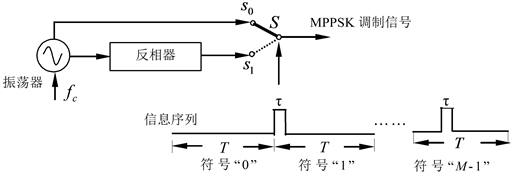Figure 1. The MPPSK modulation process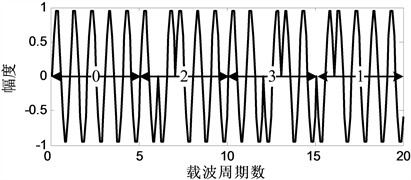Figure 2. 4PPSK modulation waveform

$\lambda =\frac{4}{{\left(\pi \left(N-\frac{{m}^{2}}{N}\right)M\right)}^{2}}{\mathrm{sin}}^{2}\left(\frac{m\left(M-1\right)K}{N}\pi \right)$ (2)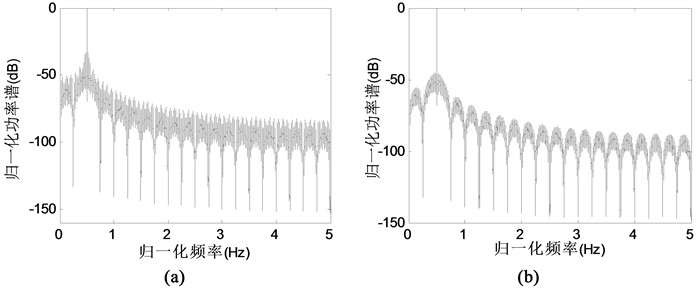Figure 3. The power spectrum of MPPSK and the power spectrum after the suppression line spectrum. (a) The power spectrum at the modulation parameters K = 2, N = 36 and M = 16; (b) The power spectrum at the modulation parameters K = 2, N = 30 and M = 16

$N=\left(M-1\right)K$ (3)

3. MPPSK系统检测与信道编码

MPPSK调制利用码元周期中不同位置的载波变化代表不同的符号，因而检测过程中确定载波变化的位置最为关键。由于信道噪声的影响，该位置有时较难精确定位，这势必会对检测性能产生较大的影响。若能找到一种方法，对位置信息不太敏感，而且能得到期望的结果，同时又可适当降低复杂度，那么将较大程度上优化MPPSK接收机，为此，本文在MPPSK系统中引入多分类SVM用以检测接收信号并进行LDPC译码。

3.1. SVM多分类

$\begin{array}{l}\underset{{\text{w}}^{pq},{b}^{pq},{\xi }^{pq}}{\text{min}}\text{\hspace{0.17em}}\text{\hspace{0.17em}}\text{\hspace{0.17em}}\text{\hspace{0.17em}}\frac{1}{2}{\left({\text{w}}^{pq}\right)}^{\text{T}}{\text{w}}^{pq}+C\underset{i}{\sum }{\xi }_{i}^{pq}\\ \text{\hspace{0.17em}}\text{\hspace{0.17em}}\text{\hspace{0.17em}}\text{\hspace{0.17em}}\text{\hspace{0.17em}}\text{\hspace{0.17em}}\text{\hspace{0.17em}}\text{\hspace{0.17em}}\text{\hspace{0.17em}}\text{\hspace{0.17em}}\text{\hspace{0.17em}}\text{\hspace{0.17em}}\text{\hspace{0.17em}}\text{\hspace{0.17em}}\text{\hspace{0.17em}}{\left({\text{w}}^{pq}\right)}^{\text{T}}\Psi \left({x}_{i}\right)+{b}^{pq}\ge 1-{\xi }_{i}^{pq},\text{\hspace{0.17em}}当\text{ }{y}_{i}=p,\\ \text{\hspace{0.17em}}\text{\hspace{0.17em}}\text{\hspace{0.17em}}\text{\hspace{0.17em}}\text{\hspace{0.17em}}\text{\hspace{0.17em}}\text{\hspace{0.17em}}\text{\hspace{0.17em}}\text{\hspace{0.17em}}\text{\hspace{0.17em}}\text{\hspace{0.17em}}\text{\hspace{0.17em}}\text{\hspace{0.17em}}\text{\hspace{0.17em}}\text{\hspace{0.17em}}{\left({\text{w}}^{pq}\right)}^{\text{T}}\Psi \left({x}_{i}\right)+{b}^{pq}\ge -1+{\xi }_{i}^{pq},\text{\hspace{0.17em}}当\text{ }{y}_{i}=q,\\ \text{\hspace{0.17em}}\text{\hspace{0.17em}}\text{\hspace{0.17em}}\text{\hspace{0.17em}}\text{\hspace{0.17em}}\text{\hspace{0.17em}}\text{\hspace{0.17em}}\text{\hspace{0.17em}}\text{\hspace{0.17em}}\text{\hspace{0.17em}}\text{\hspace{0.17em}}\text{\hspace{0.17em}}\text{\hspace{0.17em}}\text{\hspace{0.17em}}\text{\hspace{0.17em}}\text{\hspace{0.17em}}{\xi }_{i}^{pq}\ge 0.\end{array}$ (4)

3.2. MPPSK系统的非线性检测

MPPSK调制系统中非线性检测相较于传统的门限检测在M值较小的情况下可使系统性能有较大提升。采用人工神经网络反向传播(ANN-BP: Artificial Neural Network using the Back Propagation Algorithm)算法利用SIF输出的波形差异和位置特征，检测性能明显优于传统基于锁相环(PLL: Phase Locked Loop)的门限判决方法，仅劣于EBPSK门限检测 。但是，该方法并未考虑降低接收机检测的计算复杂度，将SIF输出信号采样值全部作为ANN-BP的输入，在采样率较高，即便是M值较小的情况下依然是难以承受的。若根据MPPSK调制信号的特点选择少量采样点组成特征向量作为多分类SVM输入进行检测，则可有效地降低检测的复杂度。由于MPPSK调制信号在码元周期的不同位置都有可能出现相位的跳变，这就需要在整个码元周期内选取采样点组成该特征向量，这与EBPSK调制信号有所不同。若控制选取采样点的跨度，则采用维数较少的特征向量即可完成多分类SVM的训练与测试，不仅可以较大程度上降低系统的计算复杂度，而且无需像门限检测那样在检测前先确定冲击的位置。需要指出的是，由于MPPSK调制的特点，整个符号周期内不同位置的载波变化代表着不同的符号，波形及能量的特点无法反映位置信息。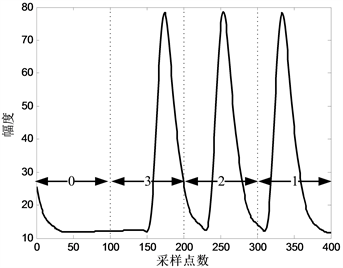Figure 4. The MPPSK modulation signal passes through the waveform after SIF

MPPSK与EBPSK接收机的唯一差别是根据波形的跳变位置进行检测，图5所示为MPPSK利用多分类SVM技术检测的结构图，其处理过程相对应的波形如图6所示。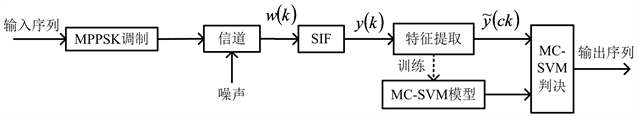Figure 5. The structure diagram of multi-classification SVM detection is applied in MPPSK system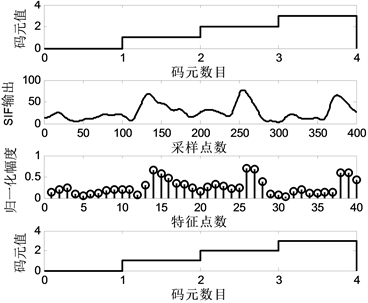Figure 6. Output waveforms detected in MPPSK based on multiclassified SVMs

4. 实验仿真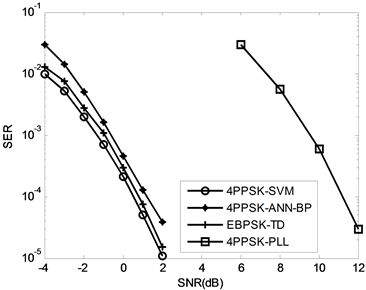Figure 7. Different methods of MPPSK detection performance and EBPSK-TD performance curve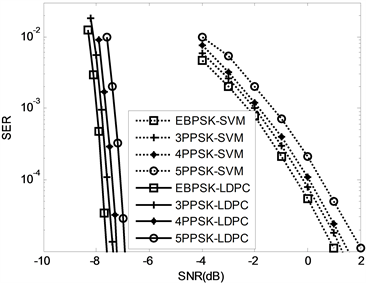Figure 8. Detection and channel encoding in the MPPSK system

5. 总结

NOTES

*通讯作者。

 吴乐南, 应鹏魁. 扩展的二元相移键控调制解调器[P]. 中国专利, ZL 201020649416.2. 2011-09-28.

 Feng, M., Wu, L.N. and Gao, P. (2012) From Special Analogous Crystal Filters to Digital Impacting Filters. Digital Signal Processing, 22, 690-696.
https://doi.org/10.1016/j.dsp.2012.02.011

 Feng, M. and Wu, L. (2009) Special Non-Linear Filter and Extension to Shannon’s Channel Capacity. Digital Signal Processing, 19, 861-873.
https://doi.org/10.1016/j.dsp.2009.03.003

 陈志敏, 贺新毅, 李靖超, 靳一. 基于高效调制的MIMO方案及性能分析[J]. 上海电机学院学报, 2018(2): 15-20 + 27.

 Wang, H.Y. and Tian, H.X. (2019) Anti-Multipath Performance Improvement of an M-Ary Position Phase Shift Keying Modulation System. Sensors, 19, 1938.
https://doi.org/10.3390/s19081938

 Yao, Y., Zhao, J.H. and Wu, L.N. (2018) Cognitive Radar Waveform Optimization Based on Kalman Filtering for target Estimation. Journal of Applied Remote Sensing, 12, Article ID: 035016.
https://doi.org/10.1117/1.JRS.12.035016

 Yao, Y., Zhao, J.H. and Wu, L.N. (2019) Adaptive Extended Binary Phase-Shift Keying Waveform Design Algorithm for Extended Target Detection. Journal of Applied Remote Sensing, 13, Article ID: 016511.
https://doi.org/10.1117/1.JRS.13.016511

 Velasquez, H.A.M. and Gordillo, A.C. (2018) EBPSK-Based Quadrature Ultra Narrow Band Modulation System. 2018 IEEE MTT-S Latin America Microwave Conference (LAMC 2018), Arequipa, 12-14 December 2018, 1-4.
https://doi.org/10.1109/LAMC.2018.8699043

 Yaom Y., Li, X. and Wu, L.N. (2018) Study of Range-Extended Target Detection Performance Based Optimized EBSPK Signals. Cluster Computing, 22, 6781-6793.
https://doi.org/10.1007/s10586-018-2650-9

 Chen, Z.M., Chen, P., Li, J.C. and Miao, P. (2018) Non-Orthogonal Multi-Carrier MIMO Communication System Using M-Ary Efficient Modulation. Digital Signal Processing, 76, 14-21.
https://doi.org/10.1016/j.dsp.2018.01.019

 Miao, P., Wu, L.N. and Chen, Z.M. (2018) An Anti-Noise Modem for Visible Light Communication Systems Using the Improved M-Ary Position Phase Shift Keying. AEU—International Journal of Electronics & Communications, 85, 126-133.
https://doi.org/10.1016/j.aeue.2017.12.039

 Chen, Z.M., Li, J.C., Chen, P. and Miao, P. (2018) Blind Source Separation for Multi-Carrier Efficient Modulations Using MIMO Antennas. In: Sun, G. and Liu, S., Eds., Lecture Notes of the Institute for Computer Sciences, Social Informatics and Telecommunications Engineering, Springer, Cham, 546-554.
https://doi.org/10.1007/978-3-319-73317-3_64

 Chen, X.Q., Song, S.B. and Zhou, W. (2014) Demodulation of a M-Ray Position Phase Shift Keying System Using Multi-Class Support Vector Machine Classification. Applied Mechanics and Materials, 687-691, 3840-3843.
https://doi.org/10.4028/www.scientific.net/AMM.687-691.3840

 Yan, Z., Ping, W., Tao, L., et al. (2018) Performance Analysis of a LDPC Coded OAM-Based UCA FSO System Exploring Linear Equalization with Channel Estimation over Atmospheric Turbulence. Optics Express, 26, 22182-22196.
https://doi.org/10.1364/OE.26.022182

 Meng, F., Chen, P., Wu, L.N. and Wang, X.B. (2018) Automatic Modulation Classification: A Deep Learning Enabled Approach. IEEE Transactions on Vehicular Technology, 67, 10760-10772.
https://doi.org/10.1109/TVT.2018.2868698

 靳一, 吴乐南, 余静, 等. MPPSK调制解调器研究[J]. 信号处理, 2012, 28(7): 917-925.

Top